# OFDM and Multi-Channel Communication Systems

## Overview

Digital communications systems require each channel to operate at a specific frequency and with a specific bandwidth. In fact, communication systems have evolved so that the largest amount of data can be communicated through a finite frequency range. In this document we will focus on the recent evolution of communications systems into using various mechanisms for effectively using the frequency spectrum. More specifically, we will describe how frequency division multiplexing (FDM) and orthogonal frequency division multiplexing (OFDM) are able to effectively utilize the frequency spectrum. In addition, we will distinguish the two and describe why OFDM systems are currently being implemented in some of the newest and most advanced communications systems.

## Frequency Division Multiplexing

Frequency division multiplexing (FDM) involves the allocation of each channel to a unique frequency range. This frequency range prescribes both the center frequency and channel width (bandwidth). Because these channels are non-overlapping, multiple users can operate concurrently simply by using different channels of the frequency domain. Below, we illustrate the frequency domain of an FDM system. Note from the diagram that each channel operates a different carrier frequency and that these channels are bandlimited to operate within a defined bandwidth.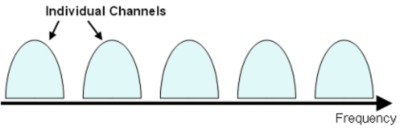While we will not discuss pulse-shape filters in depth in this document (see Pulse-Shaping Document), it is important to note that the implementation of a pulse-shaping filter allows each channel to be bandlimited to a specific frequency range.

## FDM Applications in Industry

FDM is commonly used in a variety of communications protocols including Bluetooth and cellular protocols such as GSM, TDMA, and CDMA. Bluetooth, a digital communications protocol that is utilized by cell phones, laptops, and PDA’s, is one example. It operates in the 2.4GHz unlicensed band and implements FDM by defining 79 channels from 2.402 GHz to 2.480 GHz which are spaced at 1 MHz apart. Each channel is bandlimited through the implementation of a Gaussian filter.

As second common implementation of FDM is in the Global System for Mobile Communications protocol (GSM) which is a 3G cellular communication standard. With GSM, the frequency range is divided into downlink channels from 890 - 915 MHz and the uplink channels at 935 - 960 MHz. Moreover, these frequency bands are further divided so that there are 124 channels which are spaced at 200 kHz intervals. Again, the bandwidth of each channel can be limited through the implantation of a root raised cosine filter.

## Limiting Channel Bandwidth with Pulse-Shaping

Because digital modulation involves changing characteristics of a carrier sinusoid to transmit information, filtering is an important mechanism to limit the rate at which these are altered. Sharp transitions in a modulated sinusoid result in harmonics of the carrier at higher frequencies. This can cause severe difficulty in communications system for two reasons. First, the harmonics require more power to generate. Second, an unfiltered channel requires substantial power on adjacent channels and can cause interference. We illustrate this concept by showing the frequency domain of a test channel in a simulated physical environment. In this system, each channel is spaced by 100 MHz and is 80 MHz wide. In the diagram below, we show the test channel as the white plot on the graph. The simulated physical channel is shown as the red plot. Note that test channel, centered at 1.0 GHz, shows only -20 dB of attenuation in the adjacent channel spectrum at 1.1 Ghz.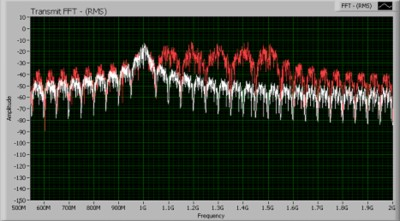As the image above illustrates, the lack of a pulse-shaping filter creates significant interference between adjacent channels. Thus, it is important to limit the bandwidth of each channel through the implementation of a pulse-shaping filter. By applying this filter, the symbol transitions are smoothed and the harmonics are eliminated. Below, we show the frequency domain of the same physical system after a pulse-shaping filter has been applied to each channel. As the image illustrates, the adjacent channel power on each channel is significantly reduced.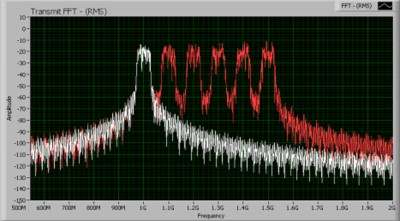Note in the diagram above that the application of filtering reduces spectral leakage into adjacent channel bands. As the image above illustrates, the interference from the test channel (1.0 GHz) has now been reduced to -70 dB after a pulse-shaping filter has been applied. Note that the bandwidth of each channel is defined exclusively by the symbol rate such that:

Bandwidth (Bw) = 2 / Symbol Rate (Rs)

Thus, by applying a pulse-shaping filter, we are able to bandlimit each channel to implement a multi-channel communications systems.

## Reducing Inter-Symbol Interference (ISI)

Not only can the pulse-shaping filters be used to eliminate interference from adjacent channels in the frequency domain, but it can also be used to eliminate interference from subsequent symbols on the same channel. Intersymbol interference (ISI) can be caused by multi-path fading as signals are transmitted over long distances and through various mediums. More specifically, this characteristic of the physical environment causes some symbols to be spread beyond their given time interval. As a result, they can interfere with the following or preceding transmitted symbols.

One solution to this problem is the application of the pulse shaping filter. By applying this filter to each symbol that is generated, we attenuate both the beginning and ending portions of the generated symbol. This reduces ISI by attenuating the last portion of each symbol which takes the longest time to reach the receiver. Below, we illustrate the implementation of a pulse shaping filter on each symbol that is generated. As the image illustrates, the maximum amplitude of the pulse-shaping filter occurs in the middle of the symbol period.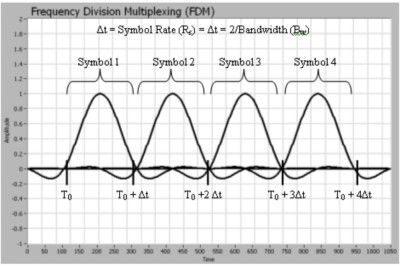As illustrated in the graph above, the peak of each symbol corresponds directly to the zero-crossing point of each subsequent symbol. In addition, the beginning and ending portions of the symbol period are attenuated. Thus, ISI is reduced by providing a pseudo-guard interval which attenuates signals from multi-path reflections. As a result, inter symbol interference (ISI) can be reduced while still limiting each channel to a specified bandwidth.

## Orthogonal Frequency Divison Multiplexing (OFDM)

OFDM is a subset of frequency division multiplexing in which a single channel utilizes multiple sub-carriers on adjacent frequencies. In addition the sub-carriers in an OFDM system are overlapping to maximize spectral efficiency. Ordinarily, overlapping adjacent channels can interfere with one another. However, sub-carriers in an OFDM system are precisely orthogonal to one another. Thus, they are able to overlap without interfering. As a result, OFDM systems are able to maximize spectral efficiency without causing adjacent channel interference. The frequency domain of an OFDM system is represented in the diagram below.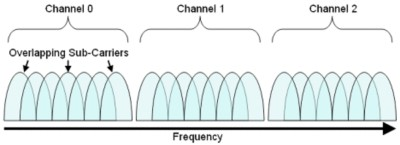Notice above that there are seven sub-carriers for each individual channel. Because the symbol rate increases as the channel bandwidth increases, this implementation allows for a greater data throughput than with an FDM system.

Orthogonality of Sub-Channel Carriers
OFDM communications systems are able to more effectively utilize the frequency spectrum through overlapping sub-carriers. These sub-carriers are able to partially overlap without interfering with adjacent sub-carriers because the maximum power of each sub-carrier corresponds directly with the minimum power of each adjacent channel. Below, we illustrate the frequency domain of an OFDM system graphically. As you can see from the figure, each sub-carrier is represented by a different peak. In addition, the peak of each sub-carrier corresponds directly with the zero crossing of all channels.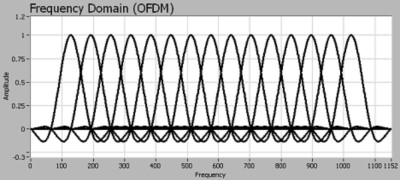Note that OFDM channels are different from bandlimited FDM channels how they apply a pulse-shaping filter. With FDM systems, a sinc-shaped pulse is applied in the time domain to shape each individual symbol and prevent ISI. With OFDM systems, a sinc-shaped pulse is applied in the frequency domain of each channel. As a result, each sub-carrier remains orthogonal to one another.
In order to use multiple sub-carriers to transmit an individual channel, an OFDM communications system must perform several steps.  These steps are described in the figure shown below.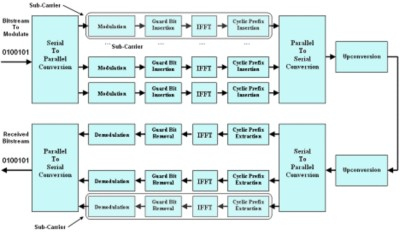Serial to Parallel Conversion
In an OFDM system, each channel can be broken into various sub-carriers. The use of sub-carriers makes optimal use out of the frequency spectrum but also requires additional processing by the transmitter and receiver. This additional processing is necessary to convert a serial bitstream into several parallel bitstreams to be divided among the individual carriers. Once the bitstream has been divided among the individual sub-carriers, each sub-carrier is modulated as if it was an individual channel before all channels are combined back together and transmitted as a whole. The receiver performs the reverse process to divide the incoming signal into appropriate sub-carriers and then demodulating these individually before reconstructing the original bitstream.

Modulation with the Inverse FFT
The modulation of data into a complex waveform occurs at the Inverse Fast Fourier Transform (IFFT) stage of the transmitter. Here, the modulation scheme can be chosen completely independently of the specific channel being used and can be chosen based on the channel requirements. In fact, it is possible for each individual sub-carrier to use a different modulation scheme. The role of the IFFT is to modulate each sub-channel onto the appropriate carrier.

Cyclic Prefix Insertion
Because wireless communications systems are susceptible to multi-path channel reflections, a cyclic prefix is added to reduce ISI. A cyclic prefix is a repetition of the first section of a symbol that is appended to the end of the symbol. In addition, it is important because it enables multi-path representations of the original signal to fade so that they do not interfere with the subsequent symbol.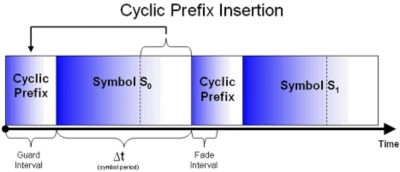Parallel to Serial Conversion
Once the cyclic prefix has been added to the sub-carrier channels, they must be transmitted as one signal. Thus, the parallel to serial conversion stage is the process of summing all sub-carriers and combining them into one signal. As a result, all sub-carriers are generated perfectly simultaneously.

Orthogonal frequency division multiplexing is commonly implemented in many emerging communications protocols because it provides several advantages over the traditional FDM approach to communications channels. More specifically, OFDM systems allow for greater spectral efficiency reduced intersymbol interference (ISI), and resilience to multi-path distortion.

Spectral Efficiency
In a traditional FDM system, each channel is spaced by about 25% of the channel width. This is done to ensure that adjacent channels do not interfere. This is illustrated in the diagram below, which shows the guard bands between individual channels.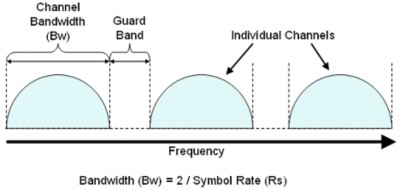Because of the requirement for guard bands, it is required to the symbol rate to allow for guard bands to exist. In general, the allowed channel bandwidth (Bw) is 2/Rs. As a result of this, the channels are able to be separated adequately.

In an OFDM system, on the other hand, the channels actually overlap. As a result, it is possible to maximize the symbol rate, and thus the throughput, for a given bandwidth. In the image below, we illustrate overlapping sub-carriers in an OFDM system. In this scenario, the channel bandwidth (Bw) approaches 1 / Rs. Thus, as the number of sub-carriers approaches infinity, OFDM systems allow for nearly double the spectral efficiency.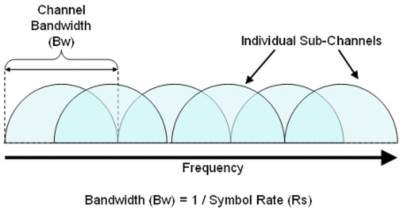Note that with an OFDM system, it is still required to have a guard band between each individual channel. However, the effective symbol rate for the combined sub-carriers is greater than if a single carrier were used instead.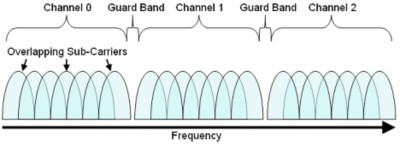Note that the effect of using overlapping orthogonal sub-carriers also requires the use of a cyclic prefix to prevent intersymbol interference (ISI). Thus, some of the advantages gained through overlapping sub-carriers are compromised. However, the spectral efficiency advantage is great enough such that greater throughput is available in an OFDM system.

Reduced Inter Symbol Interference (ISI)
In mono-carrier systems, intersymbol interference is often caused through the multi-path characteristics of a wireless communications channel. Note that when transmitting an electromagnetic wave over a long distance, the signal passes through a variety of physical mediums. As a result, the actual received signal contains the direct path signal overlaid with signal reflections of smaller amplitudes. The diagram below illustrates how, at high symbol rates, reflected signals can interfere with subsequent symbols.

In wireless systems, this creates difficulty because the received signal can be slightly distorted. In this scenario, the direct path signal arrives as expected, but slightly attenuated reflections arrive later in time. These reflections create a challenge because they interfere with subsequent symbols transmitted along the direct path. These signal reflections are typically mitigated through a pulse-shaping filter, which attenuates both the starting and ending sections of the symbol period. However, as the figure above illustrates, this problem becomes much more significant at high symbol rates. Because the reflections make up a significant percentage of the symbol period, ISI will also be substantial.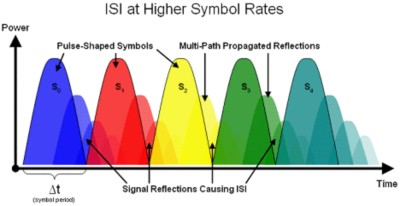OFDM systems mitigate this problem by utilizing a comparatively long symbol period. In addition, they do this without sacrificing throughput by utilizing multiple sub-carriers per channel. Below, we illustrate the time domain of OFDM symbols. Note that in an OFDM system, the symbol rate can be reduced while still achieving similar or even higher throughput.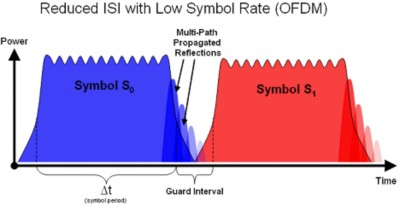Note from the illustration above that the time required for the reflections to fully attenuate is the same as before. However, by utilizing a smaller symbol rate, the signal reflections make up only a small percentage of the total symbol period. Thus, it is possible to simply add a guard interval to remove interference from reflections without significantly decreasing system throughput.

## Commercial Applications of OFDM

Several common commercial protocols, such as digital video broadcast (DVB), asymmetric digital subscriber line (ADSL), and wireless Ethernet (WiFI) implement OFDM. With WiFI, the IEEE 802.11a and IEEE 802.11g implementations specifically use OFDM techniques. With IEEE 802.11g, each channel occupies 16.25 MHz of bandwidth at the 2.4GHz frequency range. In addition, each channel is divided into 52 sub-carriers of 312.5 kHz. Together, these sub-carriers overlap to fully utilize the 16.25 MHz channel bandwidth dedicated per channel. In addition, each sub-carrier can use a unique modulation scheme. More specifically, WiFI can use BPS, QPSK, 16-QAM, or 64-QAM depending on the characteristics of the physical channel being used. One of the newest wireless internet protocols, WiMAX, also uses OFDM technology.

## WiMAX

WiMAX, or IEEE 802.16, is an internet communications protocol specifically designed to provide internet access across long wireless communications links. WiMAX boasts data throughputs of up to 75 Mbps and operates in the 2.5 GHz, 3.5GHz, and 5.8 GHz bands. In addition, it fully utilizes the OFDM approach to a communications channel. For this reason, it is more resilient to multi-path symbol interference and can be used to transmit data distances of up to 30 miles.

Each OFDM channel consists of 128 to 2048 sub-carriers and can occupy bandwidths from 1.25 MHz to 20 MHz. In addition, each of these sub-carriers is modulated using BPSK, QPSK, 16-QAM, or 64-QAM modulation, depending on the requirements of the physical channel.

In addition, WiMAX utilizes each channel’s sub-carriers in three specific ways. First, the data sub-carriers are used for data transmission. Second, WiMAX implements pilot sub-carriers which are used for channel estimation and synchronization. Finally, several sub-carriers are designated as null sub-carriers which are used as guard bands. These are arranged according to the diagram below: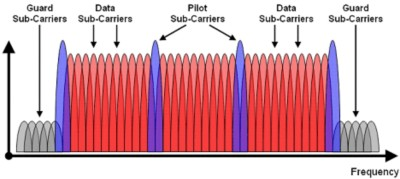Specifically, the number of sub-carriers used as null, pilot, and data purposes depends upon the specific system bandwidth being used. Each of these carriers are spaced at 11.16 kHz and the number of sub-carriers utilized determines the total system bandwidth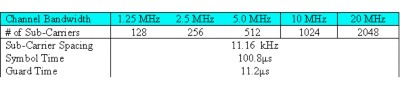As the table above illustrates, WiMAX provides a very flexible approach to communications because of the variable number of sub-carriers being used. In addition, because it uses OFDM to implement each channel, the protocol allows for data transmission over long distances with minimal multi-path distortion without sacrificing throughput.

## Conclusion and References

Orthogonal frequency division multiplexing is an important technology because many developing communications standards require the high throughput and multi-path advantages that are possible. In LabVIEW, it is possible to construct OFDM symbols with the modulation toolkit and other math and analysis functions.

1. C. Richard Johnson and William A. Sethares, Telecommunications Breakdown.
2. John G. Proakis and Masoud Salehi, Communications Systems Engineering.
3. Simon Haykin, Communications Systems.
4. B.P. Lathi, Modern Digital and analog Communications Systems.
5. John G. Proakis, Digital Communications.
6. Theodore S. Rappaport, Wireless Communications: Principles and Practice.

## Related Products

PXI Vector Signal Analyzer

PXI Vector Signal Generator

NI RF Switches
The National Instruments RF switch modules are ideal for expanding the channel count or increasing the flexibility of systems with signal bandwidths greater than 10 MHz to bandwidths as high as 26.5 GHz.

NI LabVIEW

National Instruments LabVIEW is an industry-leading graphical software tool for designing test, measurement, and automation systems.